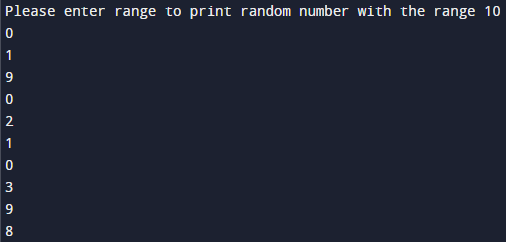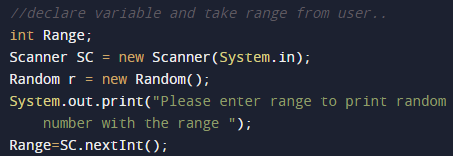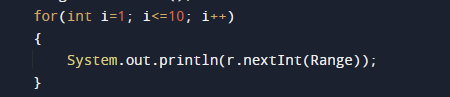# Java Program to Generate Random Numbers

In this tutorial you will learn about the Java Program to Generate Random Numbers and its application with practical example.

In this tutorial, we will learn to create a Java Program to Generate Random Numbers  using java programming.

## Prerequisites

Before starting with this tutorial, we assume that you are best aware of the following Java programming concepts:

• Java Operators.
• Basic Input and Output function in Java.
• Class and Object in Java.
• Basic Java programming.
• If-else statements in Java.
• For loop in Java.

## What is random number in java?

In Java we use Math.random() function to generate a pseudorandom number, Number created with a formula that simulates randomness.

## Java Program to Generate Random Numbers

In this program we will find random number using random() method and a for loop. We would first declared and initialized the required variables. Next, we will find find random number using random() method .Lets have a look at the code.

## Output## OutputWe can use either methods to print random numbers

In the above program, we have first declared and initialized a set variables required in the program.

• range= it will hold entered max range
• r=  it will hold random numbers
• i = for iteration

After declaring variables we initiate values.### How to generate random numbers in Java

1. first of all Import the class java.util.Random.
2. create instance of class Random, i.e., Random r = new Random()
3. generates random numbers in the range 0 to upperbound-1and within the loop we will print 10 random number within the range given by user.

Note :=> Every time we get a different output when we execute the program.

In this tutorial we have learn about the Java Program to Generate Random Numbers and its application with practical example. I hope you will like this tutorial.